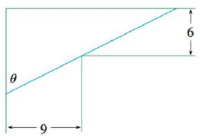Chapter 4.7, Problem 74E### Single Variable Calculus: Early Tr...

8th Edition
James Stewart
ISBN: 9781305270343

#### Solutions

Chapter
Section### Single Variable Calculus: Early Tr...

8th Edition
James Stewart
ISBN: 9781305270343
Textbook Problem

# A steel pipe is being carried down a hallway 9 ft wide. At the end of the hall there is a right-angled turn into a narrower hallway 6 ft wide. What is the length of the longest pipe that can be carried horizontally around the corner?To determine

To find: The length of the longest pipe that can be carried horizontally around the corner.

Explanation

Given:

The width of the hallway=9ft.

The width of the narrower hallway=6ft.

Calculation:

The pipe is AB=AC+CB=L1+L2

The pipe touches the hallway at the point C.

Let BCE=θ.

Hence, ACD=90θ.

As θ0 or θπ2, L.

Then, there is an angle that makes L a minimum.

For the triangle ΔACD,

cos(90θ)=9L1L1=9cosecθ

For the triangle ΔBCE,

cos(θ)=6L2L2=6secθ

Then the length of the pipe,

L=L1+L2=9cscθ+6secθ

Differentiate L with respect to θ,

L=9cosecθcotθ+6secθtanθ

For critical points, L=0

9cscθcotθ+6secθtanθ=09cscθcotθ=6secθtanθtan3θ=96

Simplify further as follows,

tanθ=(1.5)13θ=tan1(1.5)13θ=3

### Still sussing out bartleby?

Check out a sample textbook solution.

See a sample solution

#### The Solution to Your Study Problems

Bartleby provides explanations to thousands of textbook problems written by our experts, many with advanced degrees!

Get Started

#### Find more solutions based on key concepts